# In the figure to the right, the equation of the solid parabola is y=x2 - 16...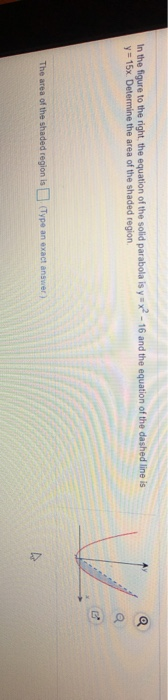In the figure to the right, the equation of the solid parabola is y=x2 - 16 and the equation of the dashed line is y = 15x Determine the area of the shaded region The area of the shaded region is Type an exact answer)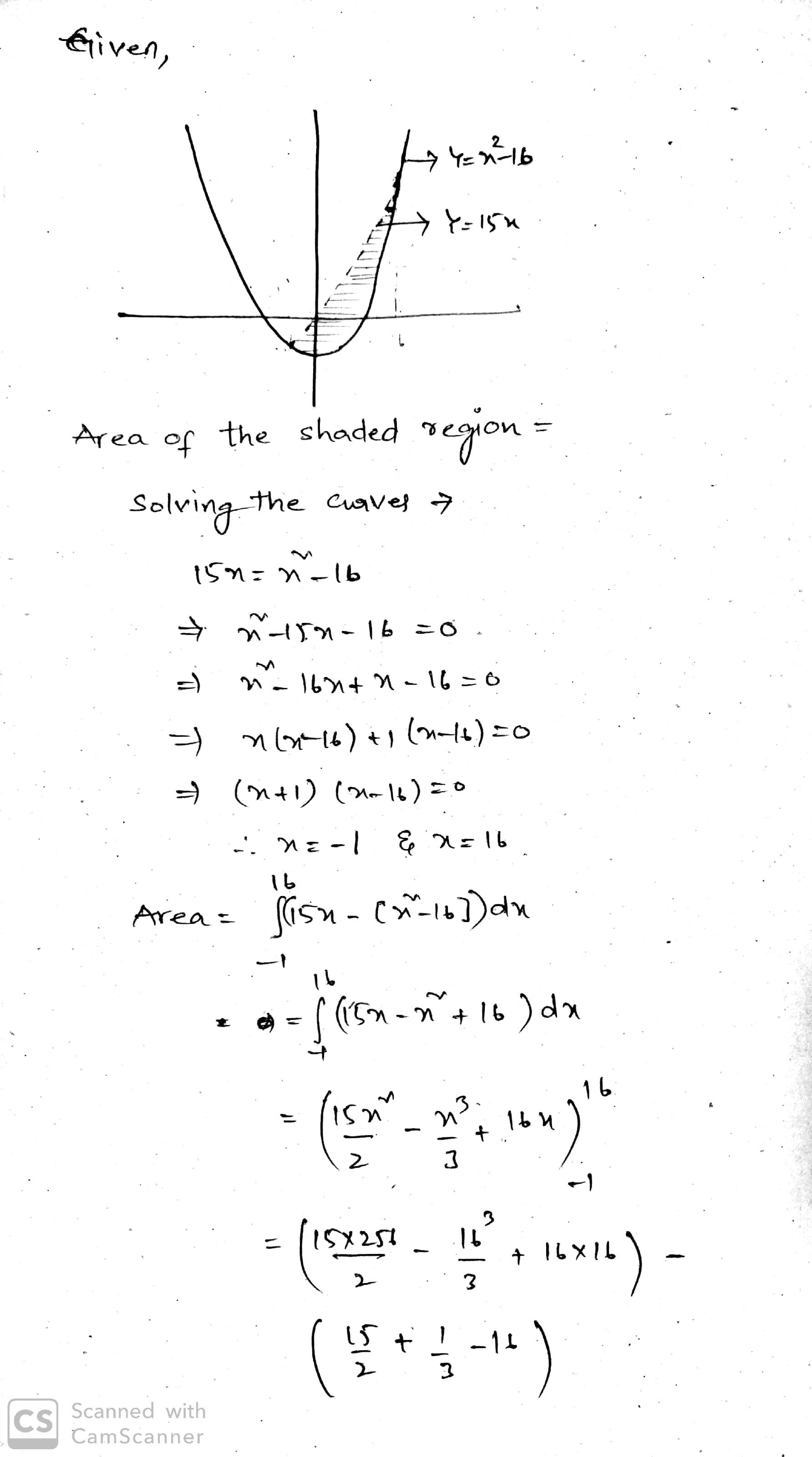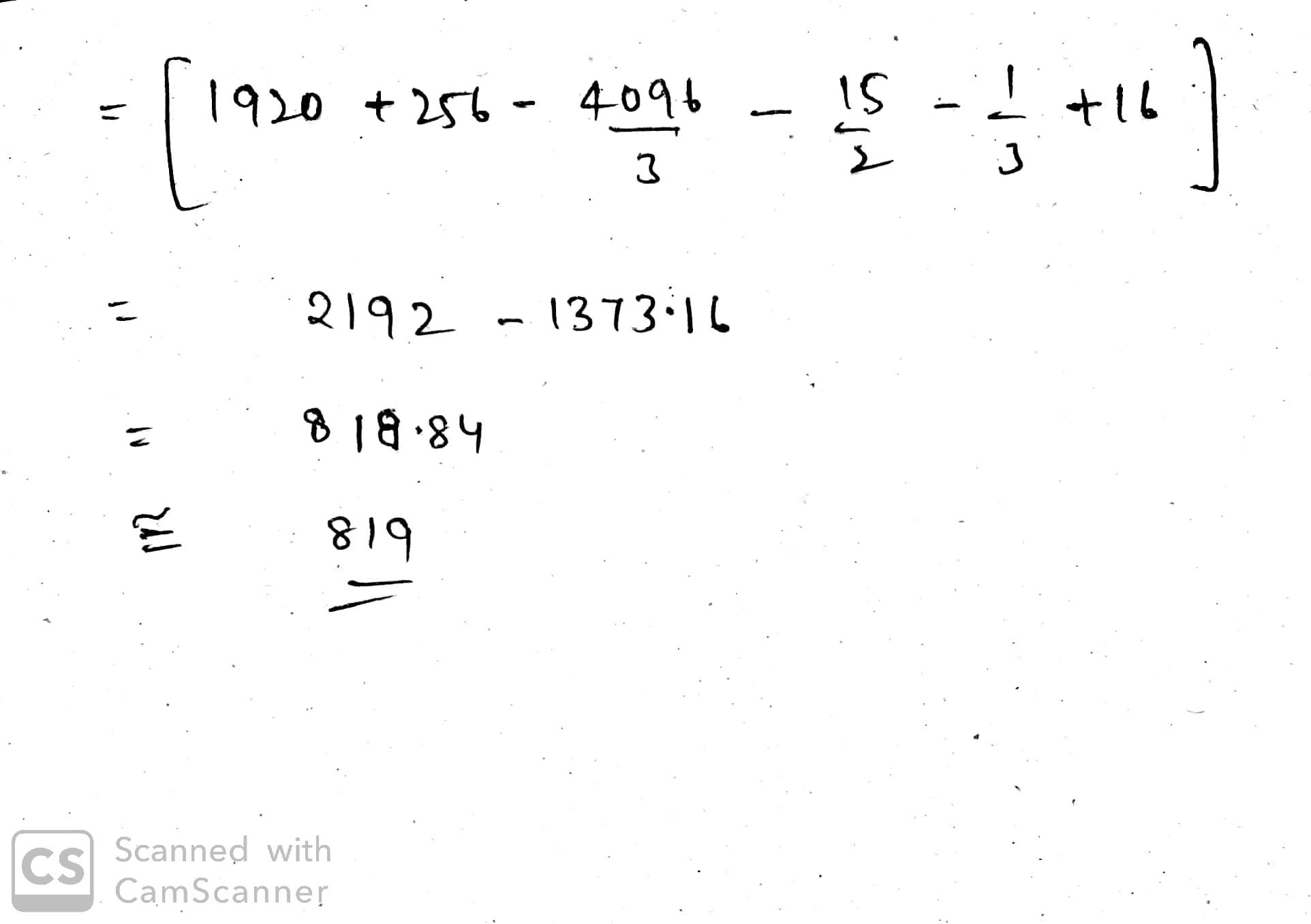##### Add Answer of: In the figure to the right, the equation of the solid parabola is y=x2 - 16...
Similar Homework Help Questions
• ### (y-2)2 In the graph to the right, the equation of the parabola is x= 2 and...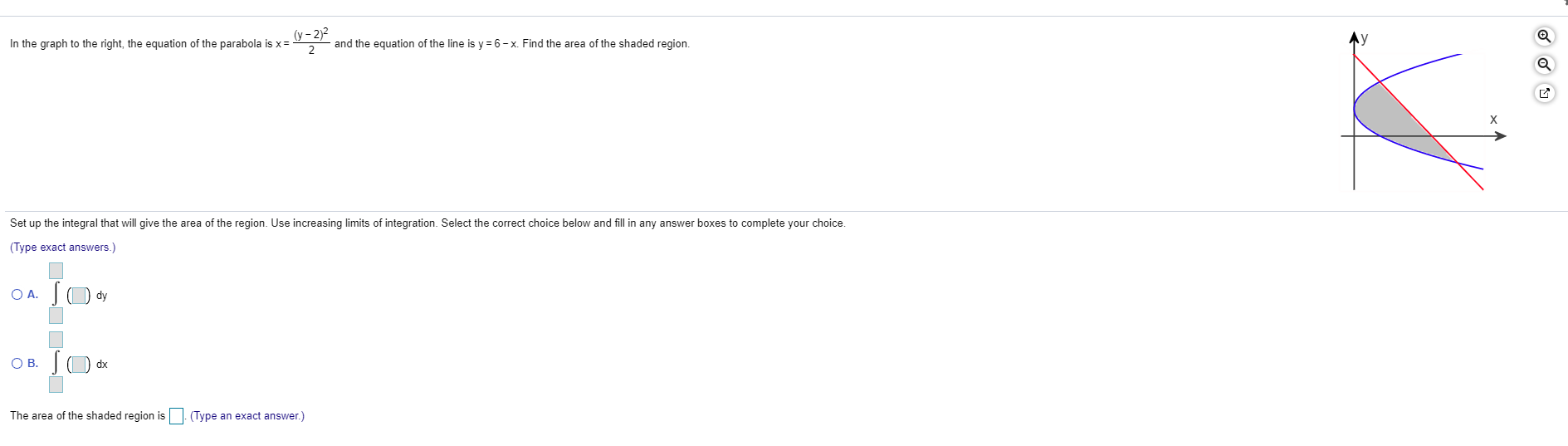(y-2)2 In the graph to the right, the equation of the parabola is x= 2 and the equation of the line is y = 6-x. Find the area of the shaded region. Set up the integral that will give the area of the region. Use increasing limits of integration. Select the correct choice below and fill in any answer boxes to complete your choice. (Type exact answers.) OA. sody OB. SO dx The area of the shaded region is (Type...

• ### In the graph to the right, the equation of the parabola is x= region (y-4)2 4...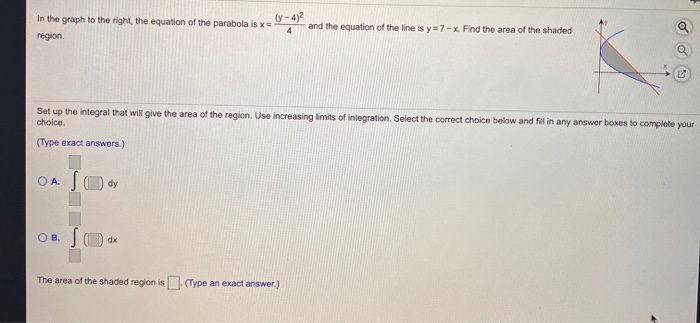In the graph to the right, the equation of the parabola is x= region (y-4)2 4 and the equation of the line is y = 7 - x. Find the area of the shaded Set up the integral that will give the area of the region. Use increasing limits of integration. Select the correct choice below and fill in any answer boxes to complete your choice (Type exact answers.) OA SO dy OB. So dx The area of the shaded...

• ### Consider the given parabolax=6y-y^2The area of the region that lies to the right of the y-axis and to the left of the parabola (the shaded region in the figure) is given by the following integral.(Turn your head clockwise and think of the region as lying below the curve x = 6y - y^2 from y = 0 to y = a = 6.)Find the area Aof the region (enter an exact answer)A=

• ### Use the shell method to find the volume of the solid generated by revolving the region bounded by the line y 5x +6 and...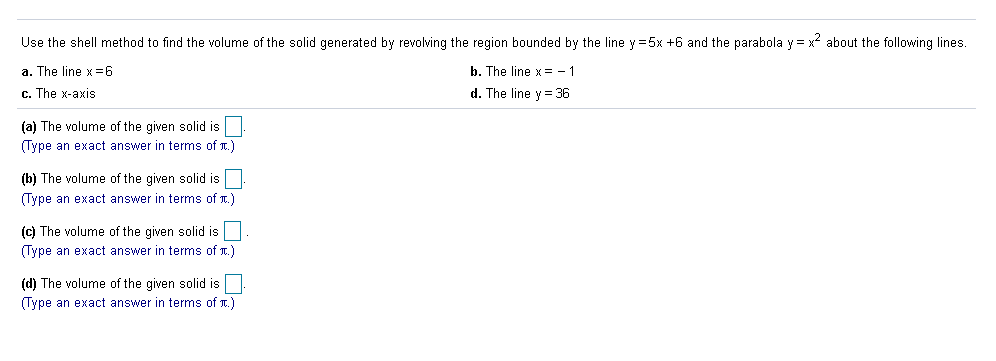Use the shell method to find the volume of the solid generated by revolving the region bounded by the line y 5x +6 and the parabola y = x about the following lines. a. The line x6 b. The line x= -1 c. The x-axis d. The line y36 (a) The volume of the given solid is (Type an exact answer in terms of .) (b) The volume of the given solid is (Type an exact answer in terms of...

• ### 1) A region is bounded by the line y = x and the parabola y = x2 - 6x + 10

1) A region is bounded by the line y = x and the parabola y = x2 - 6x + 10. What is the volume of the solid generated by revolving the region about the x-axis?

• ### Find the volume of the solid generated by revolving the shaded region about the y-axis. T...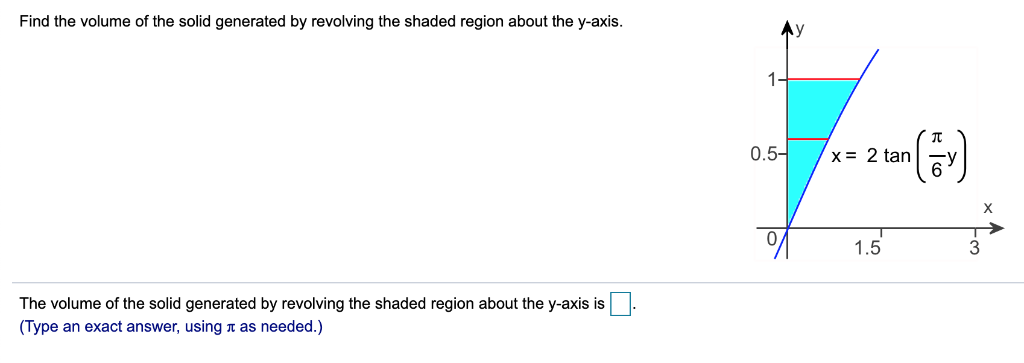Find the volume of the solid generated by revolving the shaded region about the y-axis. T 0.5 x 2 tan X 1.5 The volume of the solid generated by revolving the shaded region about the y-axis is (Type an exact answer, using n as needed.) Find the volume of the solid generated by revolving the shaded region about the y-axis. T 0.5 x 2 tan X 1.5 The volume of the solid generated by revolving the shaded region about the...

• ### Consider the following. The x y-coordinate plane is given. A horizontal line, a curve, and a...

Consider the following. The x y-coordinate plane is given. A horizontal line, a curve, and a shaded region are graphed. The horizontal line labeled f(x) = 25 crosses the y-axisat y = 25. The curve g(x) = x2 enters the window in the second quadrant, goes down and right becoming less steep, changes direction at the origin, goes up and right becoming more steep, passes through (5, 25) crossing the horizontal line, and exits the window in the first quadrant....

• ### Find the area enclosed by the parabola y2-3y+4 and the line x 2y+4 ts where the parabola and the line intersect. Gi...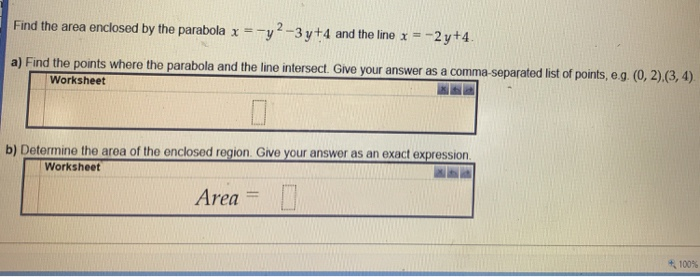Find the area enclosed by the parabola y2-3y+4 and the line x 2y+4 ts where the parabola and the line intersect. Give your answer as a comma-separated list of points, e g Worksheet b) Determine the area of the enclosed region. Give your answer as an exact expression Worksheet Area 100% Find the area enclosed by the parabola y2-3y+4 and the line x 2y+4 ts where the parabola and the line intersect. Give your answer as a comma-separated list of...

• ### 1) Problem 12 The area of the region bounded by the parabola x y-3) and the line y x is Problem 13 The base of a solid S is the parabolic region [(x.y):x s y S 1). Cross-sections perpendicular the y-...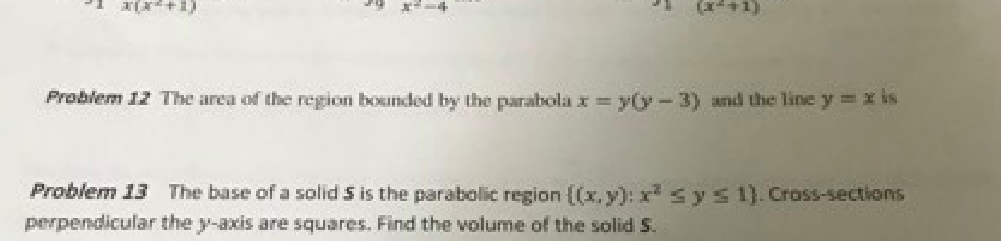1) Problem 12 The area of the region bounded by the parabola x y-3) and the line y x is Problem 13 The base of a solid S is the parabolic region [(x.y):x s y S 1). Cross-sections perpendicular the y-axis are squares. Find the volume of the solid S 1) Problem 12 The area of the region bounded by the parabola x y-3) and the line y x is Problem 13 The base of a solid S is the...

• ### all answer Sample Test 4 1575 Calculus II 1. The region bounded by the parabola y-4x-x and the x -axis is revolved about thex- axis. Find the volume of the solid. Write answer in term of π. Find...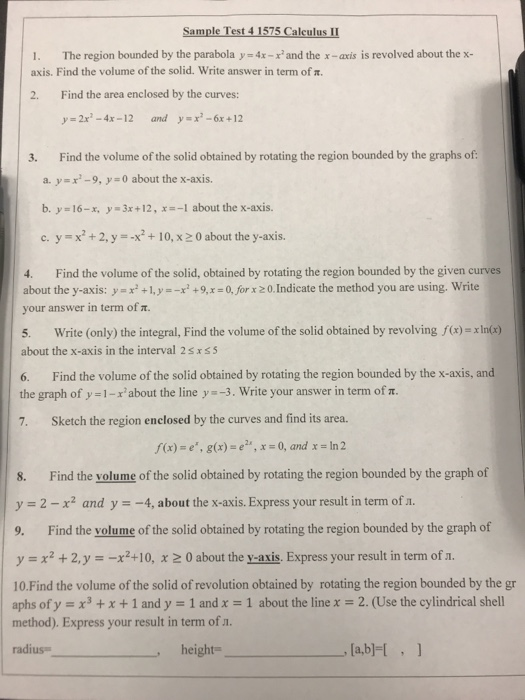all answer Sample Test 4 1575 Calculus II 1. The region bounded by the parabola y-4x-x and the x -axis is revolved about thex- axis. Find the volume of the solid. Write answer in term of π. Find the area enclosed by the curves: 2. y=2x2-4x-12 y=x2-6x+12 and 3. Find the volume of the solid obtained by rotating the region bounded by the graphs of a. y-x-9, y 0 about the x-axis. -1 about the x-axis. b. y 16-r, y-3x+...

Free Homework App